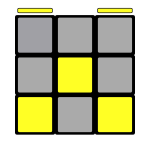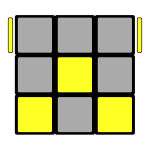# My Rubik’s Cube Solution

Post date
Categories

A number of people have been solving Rubik’s cubes at work recently, and although there are numerous guides out there I can’t seem to find one that matches my approach for every step.

## Intro: Terminology

A Rubik’s cube is made up of a number of different pieces, or cubies. There are 3 types: a corner piece (with 3 stickers), an edge piece (with 2 stickers), and a fixed centre piece. All centre pieces are fixed relative to each other, and the corner and edge pieces move around them.

Each of the faces has a name, depending on how the cube is being held: The F (front) and B (back) faces, where F faces the solver. The L (left) and R (right) faces are clear, and the U (up) and D (down) faces are top and bottom of the cube respectively.

Each face can move in 2 directions: clockwise and anti-clockwise. Clockwise is always relative to looking at the face, not from the view of the solver. A clockwise move is denoted by the face letter, so F rotates the front face clockwise. An anti-clockwise move is the letter followed by a prime symbol, such as F′. A double turn (i.e. 180°) is denoted by a letter followed by the number 2, such as F2.

## Step 1: The Cross

I choose white as the starting colour. My recommendation is to figure out the first layer (steps 1–2) without using the instructions, but I’ve created some basic ones just in case. The key is to match the colours relative to the other centre pieces. In all these isometric views below, the left-hand face of the diagram is the F face, making the right-hand face the R face.

## Step 2: First Layer Corners

Once you have the cross, fill in the corner cubies to match. First, look for an unsolved white corner in the bottom layer, and place it below where it’s meant to be in the top layer. If there aren’t any, you can just move one out of the top layer using any of these moves. As with before, the left-hand face of the picture is F.

## Step 3: Middle Layer

Now that you have the first layer complete, flip the cube upside-down so it’s on the bottom. The next step solves the four edge cubies in the middle layer. In the diagrams, the left-hand face is always F.

As with step 2, look for an edge in the top layer to move down into the middle layer. If there isn’t one, just swap one out from the middle layer.

This is where my approach differs from most guides I’ve found. The moves are 1 turn shorter, but the starting position isn’t as easy to explain. Line the edge up above the appropriate centre piece, so that the colour on top matches the centre piece. There are two possible moves, which are mirror images of each other.

If you have an edge in its correct position but the wrong orientation, you can either swap it out and back in using the moves above, or you can use the following shortcut:

## Step 4: Corner Permutations

You should now have the first two layers, with the final layer unsolved. The last layer is the reverse of the first layer — solve the corner cubes first and then the cross — but in each case, you need to sort out the permutations of the pieces separately from the orientations.

At this point, I flip the cube over again so the white face is on top, but I’ll often be glancing at the bottom face to see what stage it’s at. All the diagrams in the next steps assume you’re looking at the cube as follows:

First, pick one of the corner cubies to be in the right place. If one of them is in the correct orientation (e.g. yellow is correctly on the yellow face), then pick that one, as it’ll save time in the next step. There are only two outcomes (the first 2 are mirrors of each other). Note also that flipping the back 2 cubies is almost identical to the second permutation of 3 cubies, with a leading D move.

## Step 5: Corner Orientations

This step orients all the last layer corner cubies so that they all have the yellow face to match the centre cubes. There are only 2 algorithms, and again they are mirrors of each other.

All other possibilities can be reduced to these examples with various applications of the same algorithms, so it’s worth playing with these to see which move results in which outcome. A couple of others are quickly solved:Put the 2 correct corners at the back, then perform both algorithms from this step.Put the 2 correct corners at the back, then perform the first algorithm from the left, and the second algorithm from the right.

## Step 6: Edge Permutations

This step will repeat the permutation performed in step 4, but for the edges instead of the corners. As with all these later steps, there are a much more limited number of positions that the cube can be in at this point. Again, there are only 2 algorithms, and again they are mirror images of each other, but we will use a slightly different notation here.

Here, V refers to the vertical slice between the L and R faces. The orientation of the V moves should be considered the same as looking from the right-hand side, so a V move rotates the middle slice upwards, and a V′ move rotates the middle slice downwards. The smoothest way to perform a V move is to hold the L face still and rotate V and R together, then rotate R back.